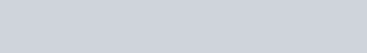Indicate the steps in the preparation of:
Question:

Indicate the steps in the preparation of:

(i) K2Cr2Ofrom chromite ore.

(ii) KMnOfrom pyrolusite ore.

Solution:

(i) Potassium dichromate $\left(\mathrm{K}_{2} \mathrm{Cr}_{2} \mathrm{O}_{7}\right)$ is prepared from chromite ore $\left(\mathrm{FeCr}_{2} \mathrm{O}_{4}\right)$ in the following steps.

Step (1): Preparation of sodium chromate

$4 \mathrm{FeCr}_{2} \mathrm{O}_{4}+16 \mathrm{NaOH}+7 \mathrm{O}_{2} \longrightarrow 8 \mathrm{Na}_{2} \mathrm{CrO}_{4}+2 \mathrm{Fe}_{2} \mathrm{O}_{3}+8 \mathrm{H}_{2} \mathrm{O}$

Step (2): Conversion of sodium chromate into sodium dichromate

$2 \mathrm{Na}_{2} \mathrm{CrO}_{4}+$ conc. $\mathrm{H}_{2} \mathrm{SO}_{4} \longrightarrow \mathrm{Na}_{2} \mathrm{Cr}_{2} \mathrm{O}_{7}+\mathrm{Na}_{2} \mathrm{SO}_{4}+\mathrm{H}_{2} \mathrm{O}$

Step(3): Conversion of sodium dichromate to potassium dichromate

$\mathrm{Na}_{2} \mathrm{Cr}_{2} \mathrm{O}_{7}+2 \mathrm{KCl} \longrightarrow \mathrm{K}_{2} \mathrm{Cr}_{2} \mathrm{O}_{7}+2 \mathrm{NaCl}$

Potassium chloride being less soluble than sodium chloride is obtained in the form of orange coloured crystals and can be removed by filtration.

The dichromate ion $\left(\mathrm{Cr}_{2} \mathrm{O}_{7}^{2-}\right)$ exists in equilibrium with chromate $\left(\mathrm{CrO}_{4}^{2-}\right)$ ion at $\mathrm{pH} 4$. However, by changing the $\mathrm{pH}$, they can be interconverted.(ii) Potassium permanganate $\left(\mathrm{KMnO}_{4}\right)$ can be prepared from pyrolusite $\left(\mathrm{MnO}_{2}\right) .$ The ore is fused with $\mathrm{KOH}$ in the presence of either atmospheric oxygen or an oxidising agent, such as $\mathrm{KNO}_{3}$ or $\mathrm{KClO}_{4}$, to give $\mathrm{K}_{2} \mathrm{MnO}_{4}$.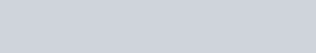The green mass can be extracted with water and then oxidized either electrolytically or by passing chlorine/ozone into the solution.

Electrolytic oxidation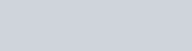At anode, manganate ions are oxidized to permanganate ions.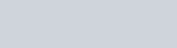Oxidation by chlorine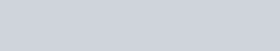Oxidation by ozone